Share

# NCERT solutions for Class 10 Mathematics chapter 10 - Circles

## Mathematics Textbook for Class 10

#### NCERT Mathematics Class 10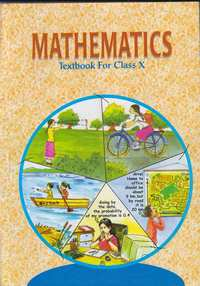## Chapter 10: Circles

Ex. 10.10Ex. 10.20

#### Chapter 10: Circles Exercise 10.10 solutions [Page 209]

Ex. 10.10 | Q 1 | Page 209

How many tangents can a circle have?

Ex. 10.10 | Q 2.1 | Page 209

Fill in the blank:

A tangent to a circle intersects it in _______ point (s).

Ex. 10.10 | Q 2.2 | Page 209

Fill in the blank:

A line intersecting a circle in two points is called a __________.

Ex. 10.10 | Q 2.3 | Page 209

Fill in the blank:

A circle can have __________ parallel tangents at the most.

Ex. 10.10 | Q 2.4 | Page 209

Fill in the blank:

The common point of a tangent to a circle and the circle is called ____

Ex. 10.10 | Q 3 | Page 209

A tangent PQ at a point P of a circle of radius 5 cm meets a line through the centre O at a point Q so that OQ = 12 cm. Length PQ is :

• 12 cm.

• 13 cm

• 8.5 cm

• sqrt119 cm test

Ex. 10.10 | Q 4 | Page 209

Draw a circle and two lines parallel to a given line such that one is a tangent and the other, a secant to the circle.

#### Chapter 10: Circles Exercise 10.20 solutions [Pages 213 - 214]

Ex. 10.20 | Q 1 | Page 213

From a point Q, the length of the tangent to a circle is 24 cm and the distance of Q from the centre is 25 cm. The radius of the circle is

• 7 cm

• 12 cm

• 15 cm

• 24.5 cm

Ex. 10.20 | Q 2 | Page 213

In the given figure, if TP and TQ are the two tangents to a circle with centre O so that ∠POQ = 110°, then ∠PTQ is equal to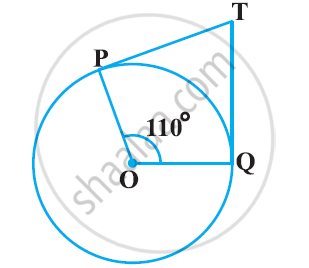• 60°

• 70°

• 80°

• 90°

Ex. 10.20 | Q 3 | Page 213

If tangents PA and PB from a point P to a circle with centre O are inclined to each other an angle of 80°, then ∠POA is equal to

• 50°

• 60°

• 70°

• 80°

Ex. 10.20 | Q 4 | Page 214

Prove that the tangents drawn at the ends of a diameter of a circle are parallel.

Ex. 10.20 | Q 5 | Page 214

Prove that the perpendicular at the point of contact to the tangent to a circle passes through the centre

Ex. 10.20 | Q 6 | Page 214

The length of a tangent from a point A at distance 5 cm from the centre of the circle is 4 cm. Find the radius of the circle.

Ex. 10.20 | Q 7 | Page 214

Two concentric circles are of radii 5 cm and 3 cm. Find the length of the chord of the larger circle which touches the smaller circle

Ex. 10.20 | Q 8 | Page 214

In the following Fig, a quadrilateral ABCD is drawn to circumscribe a circle, with centre O, in such a way that the sides AB, BC, CD and DA touch the circle at the points P, Q, R and S respectively. Prove that AB + CD = BC + DA.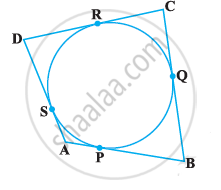Ex. 10.20 | Q 9 | Page 214

In the given figure, XY and X’Y’ are two parallel tangents to a circle with centre O and another tangent AB with point of contact C intersecting XY at A and X’Y’ at B. Prove that ∠AOB=90°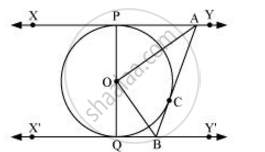Ex. 10.20 | Q 10 | Page 214

Prove that the angle between the two tangents drawn from an external point to a circle is supplementary to the angle subtended by the line segments joining the pointsof contact to the centre.

Ex. 10.20 | Q 11 | Page 214

Prove that the paralleogram circumscribing a circle, is a rhombus

Ex. 10.20 | Q 12 | Page 214

A triangle ABC is drawn to circumscribe a circle of radius 4 cm such that the segments BD and DC into which BC is divided by the point of contact D are of lengths 8 cm and 6 cm respectively (see given figure). Find the sides AB and AC.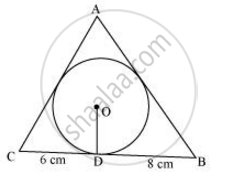Ex. 10.20 | Q 13 | Page 214

Prove that opposite sides of a quadrilateral circumscribing a circle subtend supplementary angles at the centre of the circle.

## Chapter 10: Circles

Ex. 10.10Ex. 10.20

#### NCERT Mathematics Class 10## NCERT solutions for Class 10 Mathematics chapter 10 - Circles

NCERT solutions for Class 10 Maths chapter 10 (Circles) include all questions with solution and detail explanation. This will clear students doubts about any question and improve application skills while preparing for board exams. The detailed, step-by-step solutions will help you understand the concepts better and clear your confusions, if any. Shaalaa.com has the CBSE Mathematics Textbook for Class 10 solutions in a manner that help students grasp basic concepts better and faster.

Further, we at Shaalaa.com are providing such solutions so that students can prepare for written exams. NCERT textbook solutions can be a core help for self-study and acts as a perfect self-help guidance for students.

Concepts covered in Class 10 Mathematics chapter 10 Circles are Circles Examples and Solutions, Introduction to Circles, Tangent to a Circle, Number of Tangents from a Point on a Circle.

Using NCERT Class 10 solutions Circles exercise by students are an easy way to prepare for the exams, as they involve solutions arranged chapter-wise also page wise. The questions involved in NCERT Solutions are important questions that can be asked in the final exam. Maximum students of CBSE Class 10 prefer NCERT Textbook Solutions to score more in exam.

Get the free view of chapter 10 Circles Class 10 extra questions for Maths and can use Shaalaa.com to keep it handy for your exam preparation

S